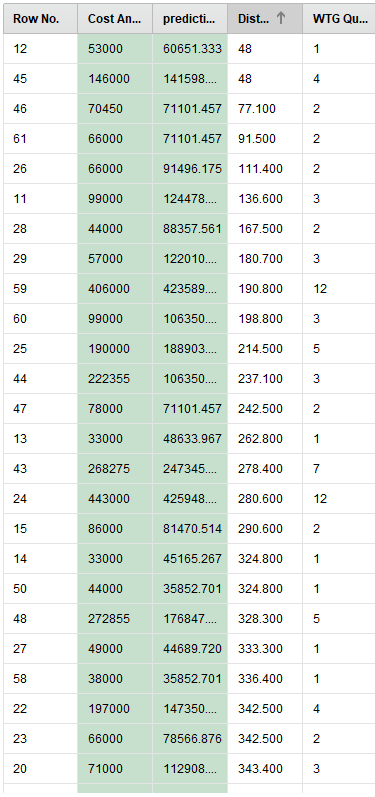# Question regarding linear regression model output

Member Posts: 3Learner I
in Help
Hi RapidMiner Community
I tried to make a linear regression model and tried testing the performance of the model through cross validation. The output is a linear function:
```- 31.472 * Distance in kilometers
+ 34850.105 * WTG Quantity
+ 15042.279```
The model performs very well at predicting the cost that I am seeking. However, the output in the predict column in cross validation does not match the variables in the overall function. If I insert a given distance and a given WTG quantity in the function, the output is not the same as the predict(variable).If the first values are inserted into the output function in Row No. 12, with a distance of 48 and WTG quantity of 1, the output is 48,381.73. However, the model predicts 60,651.
Does anyone know how the 'predict' column in cross-validation works when it predicts based on the variables that are set up. and why it is different from the result of the linear regression model?

Kind regards
Aksel

• Moderator, RapidMiner Certified Analyst, Member Posts: 1,195Unicorn
Solution Accepted
Hi @akselerator,

It is because during the 10 fold cross validation, RapidMiner produce 10 different models with each fold of data.
However, the model delivered at the output is built with the entire dataset.
Thus the models of each cross validation fold are different from the "production" model (the equation you showed).
That's why you can not retrieve the prediction of one or several models of the cross validation with the equation of the "production model".

I hope it is clear

Regards,

Lionel

• Member Posts: 3Learner I
Hi @lionelderkrikor

Thank you so much. It makes much sense.

Kind regards,
Aksel
• Moderator, RapidMiner Certified Analyst, Member Posts: 1,195Unicorn
You're welcome, Aksel !

Regards,

Lionel